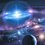# Number Theory

This hypothesis (or theory)

There are no solutions for the following equation,

${ n }^{ p }={ m }^{ p }-1$

OR

${ n }^{ p }={ m }^{ p }-2$

Where $p$ is a prime number,

$n,m$ are positive integers and must be bigger than 1.Note by Luke Zhang
5 years, 9 months ago

This discussion board is a place to discuss our Daily Challenges and the math and science related to those challenges. Explanations are more than just a solution — they should explain the steps and thinking strategies that you used to obtain the solution. Comments should further the discussion of math and science.

When posting on Brilliant:

• Use the emojis to react to an explanation, whether you're congratulating a job well done , or just really confused .
• Ask specific questions about the challenge or the steps in somebody's explanation. Well-posed questions can add a lot to the discussion, but posting "I don't understand!" doesn't help anyone.
• Try to contribute something new to the discussion, whether it is an extension, generalization or other idea related to the challenge.

MarkdownAppears as
*italics* or _italics_ italics
**bold** or __bold__ bold
- bulleted- list
• bulleted
• list
1. numbered2. list
1. numbered
2. list
Note: you must add a full line of space before and after lists for them to show up correctly
paragraph 1paragraph 2

paragraph 1

paragraph 2

[example link](https://brilliant.org)example link
> This is a quote
This is a quote
    # I indented these lines
# 4 spaces, and now they show
# up as a code block.

print "hello world"
# I indented these lines
# 4 spaces, and now they show
# up as a code block.

print "hello world"
MathAppears as
Remember to wrap math in $$ ... $$ or $ ... $ to ensure proper formatting.
2 \times 3 $2 \times 3$
2^{34} $2^{34}$
a_{i-1} $a_{i-1}$
\frac{2}{3} $\frac{2}{3}$
\sqrt{2} $\sqrt{2}$
\sum_{i=1}^3 $\sum_{i=1}^3$
\sin \theta $\sin \theta$
\boxed{123} $\boxed{123}$

Sort by:

Isn't this obvious, for any natural number $p > 2$?

The difference of consecutive powers is at least $2 ^ p - 1$.

Staff - 5 years, 9 months ago

Wait waaaaa?????????? I just gave a comment on it. Not proved it.

And u must state that $m$ and $n$ are not $0,1,-1$

- 5 years, 9 months ago

No need to state that because u already said that it must be greater than 1

- 5 years, 9 months ago

I asked whether it is true for n^p=m^p-3 or 4 .............

- 5 years, 9 months ago

I'll give you a clue, then you can figure out for yourself:

$m^{p}-n^{p}=k$

The difference between $2$ consecutive powers are always more than $k$.

What must $k$ be?

- 5 years, 9 months ago

k=k

- 5 years, 9 months ago

Wooaaahhhh very insightful! Illuminati!

- 5 years, 9 months ago

I understood your theorem. Very good and thanks for making me realize my mistake

- 5 years, 9 months ago

Can this also be correct instead of 2 if we keep 3,4,5..........

- 5 years, 9 months ago

im sorry. Im not good

- 5 years, 9 months ago

I was actually starting to think if this was a troll...

- 5 years, 9 months ago

Dood, im like 10 times worse than u lol.

- 5 years, 9 months ago

I dun even know calculus

- 5 years, 9 months ago

K noted.

- 5 years, 9 months ago